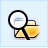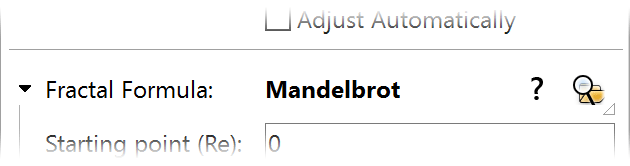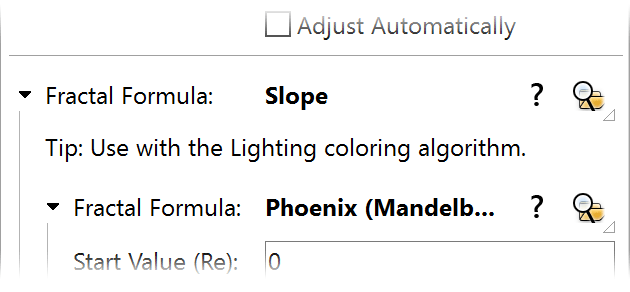# Example 1 - Formula plug-ins

 Tip: This example assumes that you are familiar with Ultra Fractal basics as explained in the tutorials.

As a first example of how plug-ins work in practice, let's take a look at some of the standard plug-ins that come with Ultra Fractal.

 First, use the browser to open the Default parameter set in Examples.upr.Click the Browse button in the Formula tab of the Layer Properties tool window to select Plug-In Formula in Standard.ufm.

Plug-In Formula is a "skeleton" formula that lets a plug-in implement the actual fractal formula behavior. It has just one plug-in parameter called Fractal Formula which is set to the Mandelbrot plug-in by default:The Mandelbrot plug-in has its own parameters like Starting point, which appear grouped with the plug-in parameter. Let's select a different formula plug-in.Click the Browse button for the Fractal Formula parameter to select a new plug-in. This opens the browser in plug-in mode, so it shows only those plug-ins that are compatible with this plug-in parameter. Locate the Newton plug-in in Standard.ulb and click OK.

Now, a Newton fractal appears instead of a Mandelbrot fractal. Formula authors could make a formula plug-in that works with Plug-In Formula to create a different fractal, without having to modify Plug-In Formula itself. Of course, this doesn't really do anything new, since you could also just write a new fractal formula. But it does make the formula behavior available in a generic form.Click the Browse button for the Fractal Formula parameter again, and this time, select the Slope plug-in.Briefly go to the Outside tab of the Layer Properties and click the Browse button there to select the Lighting coloring algorithm. Then go back to the Formula tab again.

The combination of the Slope formula plug-in and the Lighting coloring algorithm creates a 3D lighting effect. Previously, there were three different Slope formulas for Mandelbrot, Julia, and Newton fractals (these are still in Standard.ufm). Each of these formulas duplicates the code for the lighting effect; the only different code is the where the actual fractal formula calculation is done. Adding a new fractal type could only be done by creating yet another variation of this formula.

However, now the Slope formula plug-in contains another plug-in parameter that enables you to select the internal fractal formula. You can select any formula plug-in, just like with Plug-In Formula.Click the Browse button for the Fractal Formula parameter inside the Slope plug-in (which currently is set to Mandelbrot) and select Phoenix (Mandelbrot) instead.Suddenly, we have a Slope formula that works with any fractal type. New fractal types can be added simply by writing them as a formula plug-in, which makes them available for Plug-In Formula, Slope, or any other place where a fractal formula can be inserted.